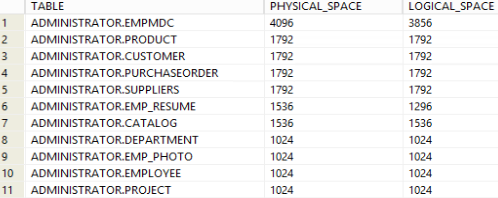## List tables by their size in Db2 database

Article for:

Query below returns tables in a database with space they use ordered from the ones using most.

## Query

``````select tabschema || '.' || tabname as table,
decimal((data_object_p_size + index_object_p_size +
long_object_p_size + lob_object_p_size +
xml_object_p_size)/1024,10,2) as physical_space,
decimal((data_object_l_size + index_object_l_size +
long_object_l_size + lob_object_l_size +
xml_object_l_size)/1024,10,2) as logical_space
where tabschema not like 'SYS%'
order by physical_space desc
``````

## Columns

• table - table name with schema name
• physical_space - disk space physically allocated for the table in MB
• logical_space - disk space logically allocated for table in MB. The logical size is the amount of space that the table knows about.

## Rows

• One row represents one table in a database
• Scope of rows: all tables in a database
• Ordered by table physical size, from largest to smallest

## Sample results